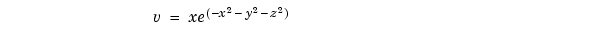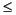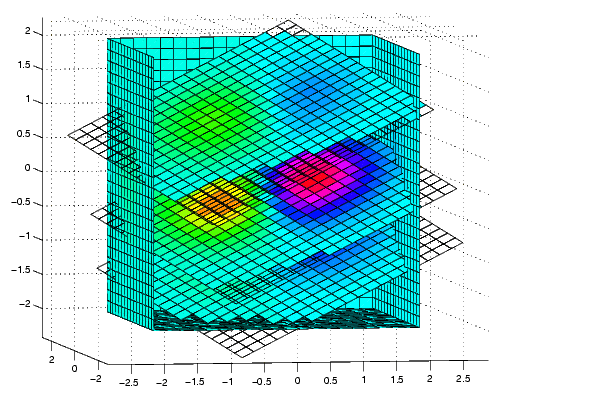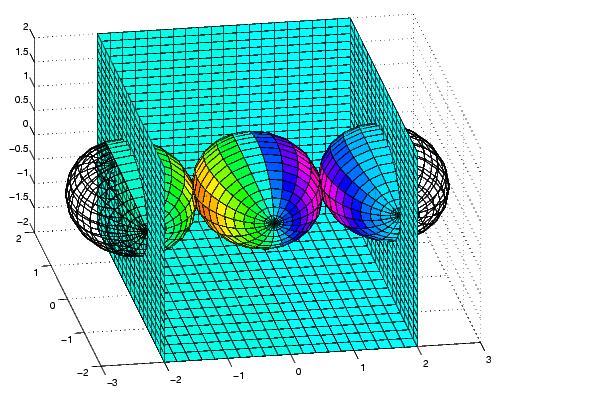MATLAB Function Referenceslice

Volumetric slice plot

Syntax

• ```slice(V,sx,sy,sz)
slice(X,Y,Z,V,sx,sy,sz)
slice(V,XI,YI,ZI)
slice(X,Y,Z,V,XI,YI,ZI)
slice(...,'`method`')
h = slice(...)
```

Description

`slice` displays orthogonal slice planes through volumetric data.

```slice(V,sx,sy,sz) ``` draws slices along the x, y, z directions in the volume `V` at the points in the vectors `sx`, `sy`, and `sz`. `V` is an m-by-n-by-p volume array containing data values at the default location `X = 1:n,` `Y = 1:m,` `Z =` `1:p`. Each element in the vectors `sx`, `sy`, and `sz` defines a slice plane in the x-, y-, or z-axis direction.

```slice(X,Y,Z,V,sx,sy,sz) ``` draws slices of the volume `V`. `X`, `Y`, and `Z` are three-dimensional arrays specifying the coordinates for `V`. `X`, `Y`, and `Z` must be monotonic and orthogonally spaced (as if produced by the function `meshgrid`). The color at each point is determined by 3-D interpolation into the volume `V`.

```slice(V,XI,YI,ZI) ``` draws data in the volume `V` for the slices defined by `XI`, `YI`, and `ZI`. `XI`, `YI`, and `ZI` are matrices that define a surface, and the volume is evaluated at the surface points. `XI`, `YI`, and `ZI` must all be the same size.

```slice(X,Y,Z,V,XI,YI,ZI) ``` draws slices through the volume `V` along the surface defined by the arrays `XI`, `YI`, `ZI`.

```slice(...,'method') ``` specifies the interpolation method. `'``method``'` is `'linear'`, `'cubic'`, or `'nearest'`.

• `linear` specifies trilinear interpolation (the default).
• `cubic` specifies tricubic interpolation.
• `nearest` specifies nearest neighbor interpolation.

```h = slice(...) ``` returns a vector of handles to surface graphics objects.

Remarks

The color drawn at each point is determined by interpolation into the volume `V`.

Examples

Visualize the functionover the range -2x2, -2y2, - 2z2:

• ```[x,y,z] = `meshgrid`(-2:.2:2,-2:.25:2,-2:.16:2);
v = x.`*`exp(-x.^2-y.^2-z.^2);
xslice = [-1.2,.8,2]; yslice = 2; zslice = [-2,0];
`slice`(x,y,z,v,xslice,yslice,zslice)
colormap hsv```

Slicing At Arbitrary Angles

You can also create slices that are oriented in arbitrary planes. To do this,

• Create a slice surface in the domain of the volume (`surf`, `linspace`).
• Orient this surface with respect the the axes (`rotate`).
• Get the `XData`, `YData`, and` ZData` of the surface (`get`).
• Use this data to draw the slice plane within the volume.

For example, these statements slice the volume in the first example with a rotated plane. Placing these commands within a for loop "passes" the plane through the volume along the z-axis.

• ```for i = -2:.5:2
hsp = surf(linspace(-2,2,20),linspace(-2,2,20),zeros(20)+i);
rotate(hsp,[1,-1,1],30)
xd = get(hsp,'XData');
yd = get(hsp,'YData');
zd = get(hsp,'ZData');
delete(hsp)
slice(x,y,z,v,[-2,2],2,-2) % Draw some volume boundaries
hold on
slice(x,y,z,v,xd,yd,zd)
hold off
axis tight
view(-5,10)
drawnow
end
```

The following picture illustrates three positions of the same slice surface as it passes through the volume.

• ``````

Slicing with a Nonplanar Surface

You can slice the volume with any surface. This example probes the volume created in the previous example by passing a spherical slice surface through the volume.

• ```[xsp,ysp,zsp] = sphere;
slice(x,y,z,v,[-2,2],2,-2)  % Draw some volume boundaries

for i = -3:.2:3
hsp = surface(xsp+i,ysp,zsp);
rotate(hsp,[1 0 0],90)
xd = get(hsp,'XData');
yd = get(hsp,'YData');
zd = get(hsp,'ZData');
delete(hsp)
hold on
hslicer = slice(x,y,z,v,xd,yd,zd);
axis tight
xlim([-3,3])
view(-10,35)
drawnow
delete(hslicer)
hold off
end
```

The following picture illustrates three positions of the spherical slice surface as it passes through the volume.See Also

`interp3`, `meshgrid`

Volume Visualization for related functions

Exploring Volumes with Slice Planes for more examples.size (serial) smooth3